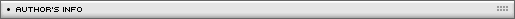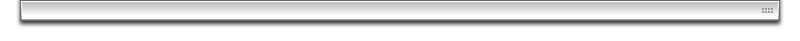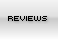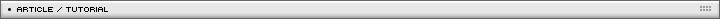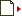Game creation tutorial Page 1/1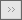[ February 26, 2007 ] Emanuele Feronato This is the second part of a long step-by-step article that will guide you through the entire process of creating a Flash game including input management, basic physics, collision detection and a lot more!

Welcome to the second step.

As you can see, now I can move the hero in a quite funny way.

Now, it's time to introduce some (deadly) obstacles.

The bounds

Every game area has bounds, so I created a movieclip called "bounds" and instanced as "wall".

My hero can't touch any wall, so the actionscript will be:

yspeed = 0;
xspeed = 0;
wind = 0.00;
power = 0.65;
gravity = 0.1;
upconstant = 0.75;
friction = 0.99;
}
onClipEvent (enterFrame) {
if (Key.isDown(Key.LEFT)) {
xspeed = xspeed-power;
}
if (Key.isDown(Key.RIGHT)) {
xspeed = xspeed+power;
}
if (Key.isDown(Key.UP)) {
yspeed = yspeed-power*upconstant;
}
if (Key.isDown(Key.DOWN)) {
yspeed = yspeed+power*upconstant;
}
xspeed = (xspeed+wind)*friction;
yspeed = yspeed+gravity;
_y = _y+yspeed;
_x = _x+xspeed;
_rotation = _rotation+xspeed;
if (_root.wall.hitTest(_x, _y, true)) {
xspeed = 0;
yspeed = 0;
_x = 120;
_y = 120;
}
}

What happened?
I added lines 28 to 33, let's see how do they work.

Line 28: I perform a hit test between the hero and the movieclip called "wall". The _x and _y means that I am checking the collision only on the _x and _y attributes of the hero - its center -
Why am I doing it this way? Because I think the game would be too frustrating if I should check the collision between any pixel of the hero and the game bounds. Moreover, the smallest the collision area (1 pixel in this case), the more complicate and creative the bounds you can draw to make the player move around it.
Lines 29 to 32 simply "reset" hero's position in case of death.

The coin - 1st attempt

Now I want my hero to be able to collect coins or similar things, so I created a new movieclip named and instanced as "coin".

New actionscript is:

yspeed = 0;
xspeed = 0;
wind = 0.00;
power = 0.65;
gravity = 0.1;
upconstant = 0.75;
friction = 0.99;
}
onClipEvent (enterFrame) {
if (Key.isDown(Key.LEFT)) {
xspeed = xspeed-power;
}
if (Key.isDown(Key.RIGHT)) {
xspeed = xspeed+power;
}
if (Key.isDown(Key.UP)) {
yspeed = yspeed-power*upconstant;
}
if (Key.isDown(Key.DOWN)) {
yspeed = yspeed+power*upconstant;
}
xspeed = (xspeed+wind)*friction;
yspeed = yspeed+gravity;
_y = _y+yspeed;
_x = _x+xspeed;
_rotation = _rotation+xspeed;
if (_root.wall.hitTest(_x, _y, true)) {
xspeed = 0;
yspeed = 0;
_x = 120;
_y = 120;
}
if (_root.coin.hitTest(_x, _y, true)) {
_root.coin._x = Math.random()*400+50;
}
}

Look at lines 34-36.
Line 34 performs basically the same test as I did for the bounds, line 35 simply moves the coin to another position once you collect it.
Wonderful.
Now try to collect the coin.

It looks hard and buggy, isn't it?
That's why I am performing the hit test only with the center of the hero, I mean only with one pixel.
While this method was good for the bounds, now it looks awful.

The coin - 2nd attempt

I am trying a different approach, changing line 34 with

if (_root.coin.hitTest(this)) {

I have extended the hit test to the entire shape.

Try to collect the coin now...

Now it's too easy!!
You can collect coins just staying close to them. Why?
Because Flash performs that hit test basing on a rectangular shape built around the hero's shape.
Just imagine a bounding box. Everything you hit with the bounding box returns a true value.
While the 1st attempt looked hard and buggy, this one looks easy and buggy. Keyword: buggy.

The coin - 3rd attempt

Are you about to give up? No?
Well, I have the solution.
Just create a new movieclip called "hero_hit" and insert into the hero's movieclip, at its center. That's the small blue square you notice on the movie.
Now perform the hit test on the hero_hit movieclip instead of the hero itself.
You can obvioulsy change the hero_hit size according to the feeling you want to give to your game.
The smaller the size, the hardes to collect coins.
Final actionscript is:

yspeed = 0;
xspeed = 0;
wind = 0.00;
power = 0.65;
gravity = 0.1;
upconstant = 0.75;
friction = 0.99;
}
onClipEvent (enterFrame) {
if (Key.isDown(Key.LEFT)) {
xspeed = xspeed-power;
}
if (Key.isDown(Key.RIGHT)) {
xspeed = xspeed+power;
}
if (Key.isDown(Key.UP)) {
yspeed = yspeed-power*upconstant;
}
if (Key.isDown(Key.DOWN)) {
yspeed = yspeed+power*upconstant;
}
xspeed = (xspeed+wind)*friction;
yspeed = yspeed+gravity;
_y = _y+yspeed;
_x = _x+xspeed;
_rotation = _rotation+xspeed;
if (_root.wall.hitTest(_x, _y, true)) {
xspeed = 0;
yspeed = 0;
_x = 120;
_y = 120;
}
if (_root.coin.hitTest(this.hero_hit)) {
_root.coin._x = Math.random()*400+50;
}
}

As you can see, the hit test at line 34 checks collision between the coin movieclip and the hero_hit movieclip

And here ends the second part..